﻿ Analysis of Rectangular Plate with Opening by Finite Difference Method

### Analysis of Rectangular Plate with Opening by Finite Difference Method

Md. Roknuzzaman, Md. Belal Hossain, Md. Rashedul Haque, Dr. Tarif Uddin Ahmed

American Journal of Civil Engineering and Architecture

## Analysis of Rectangular Plate with Opening by Finite Difference Method

Md. Roknuzzaman1,, Md. Belal Hossain1, Md. Rashedul Haque1, Dr. Tarif Uddin Ahmed2

1Department of Civil Engineering, Hajee Mohammad Danesh Science and Technology University, Dinajpur, Bangladesh

2Department of Civil Engineering, Rajshahi University of Engineering & Technology, Rajshahi, Bangladesh

### Abstract

Steel plates are commonly used to support lateral or vertical loads. Before the design of such a plate, analysis is performed to check the stability of plate for the proposed load. There are several methods for this analysis. In this research, a finite difference method (FDM) is proposed to analyze a rectangular steel plate. The plate is considered to be subjected to an arbitrary transverse uniformly distributed loading and is considered to be clamped at the two opposite edges and free at the other two edges. At first the plate is analyzed considering it to be completely solid and then it was re-analyzed considering a rectangular opening at its centre. The ordinary finite difference method is used to solve the governing differential equation of the plate deflection. The proposed method can be easily programmed to readily apply on a plate problem. The work covers the determination of displacement components at different points of the plate and checking the result by software (STAAD.Pro) analysis.

• Md. Roknuzzaman, Md. Belal Hossain, Md. Rashedul Haque, Dr. Tarif Uddin Ahmed. Analysis of Rectangular Plate with Opening by Finite Difference Method. American Journal of Civil Engineering and Architecture. Vol. 3, No. 5, 2015, pp 165-173. http://pubs.sciepub.com/ajcea/3/5/3
• Roknuzzaman, Md., et al. "Analysis of Rectangular Plate with Opening by Finite Difference Method." American Journal of Civil Engineering and Architecture 3.5 (2015): 165-173.
• Roknuzzaman, M. , Hossain, M. B. , Haque, M. R. , & Ahmed, D. T. U. (2015). Analysis of Rectangular Plate with Opening by Finite Difference Method. American Journal of Civil Engineering and Architecture, 3(5), 165-173.
• Roknuzzaman, Md., Md. Belal Hossain, Md. Rashedul Haque, and Dr. Tarif Uddin Ahmed. "Analysis of Rectangular Plate with Opening by Finite Difference Method." American Journal of Civil Engineering and Architecture 3, no. 5 (2015): 165-173.

 Import into BibTeX Import into EndNote Import into RefMan Import into RefWorks

123
Prev Next

### 1. Introduction

Analysis or solution of a plate means the determination of displacement, moment, stress etc. at various points of it. Plate analysis is very important to ensure the stability of the plate to resist the design load.

A number of research works were performed on the solution of plate problems by various classical, numerical and engineering methods in the past years. Plate bending and its characteristics were analyzed by various researchers using numerous techniques. Cheung M.S., Chan M.Y.T.  used finite strip method for static and dynamic analysis of plates. On the other hand, Fernandes G.R., Venturini W.S.  applied boundary element method for analysis of plate bending. Also, recently, Baltacioglu A.K., Akgoz, B. and Civalek, O.  made an analysis of nonlinear static response of laminated composite plates where they used discrete singular convolution method.

Problems of uniformly loaded simply supported square plates were solved by Leissa, A. W. and Niedenfuhr, F. W. . They solved four problems with different types of loading. They used the method of point matching to solve the problems and compared the results with other known solutions.

G. Aksu and R. Ali  examined free vibration characteristics of rectangular stiffened plates having a single stiffener by using the finite difference method. They used a variational technique to minimize the total energy of the stiffened plate and then replaced the derivatives appearing in the energy functional by finite difference equations.

J. N. Reddy and R. Gera  used an improved finite-difference analysis to analyze isotropic as well as orthotropic rectangular plates under various loadings and edge conditions.

Vitor M. A. Leitao  presented a meshless method for the analysis of bending of thin plates based on the use of radial basis functions to build an approximation of the general solution of the partial differential equations governing the Kirchhoff plate bending problem.

Mohd. Yusoff Bin Salleh  made a finite difference simulation of a flexible rectangular plate structure.

Recently, Ali Ergün and Nahit Kumbasar  attempted to use Improved Finite Difference Scheme (IFDS) for thin plates. They applied this method on four problems each having different boundary conditions and compared the result with ordinary FDM, FEM (Finite Element Method) and exact solution by Timoshenko’s approach. They found the IFDS to be satisfactory in application for plate problems.

In most of the cases, researchers carried out their analysis using a specific technique and then compared their results with other available known solutions to check validity of their analysis. In the present work, a rectangular steel plate with a central opening is analyzed using finite difference method and the results is compared with a finite element based STAAD.Pro software pakage solution.Download asVeiw figureFigures index
Figure 1. Laterally loaded rectangular plate

### 2. Classical Plate Theory

An exact stress analysis of a thin plate subjected to loads acting normal to its surface requires solution of the differential equations of three-dimensional elasticity. In most cases, however, such an approach would encounter insurmountable mathematical difficulties. Yet, for the vast majority of technical applications Kirchhoff’s classical theory of thin plates yields sufficiently accurate results without the need of carrying out a full three-dimensional stress analysis. It is formulated in terms of transverse deflections w(x, y) for which the governing differential equation is of fourth order, requiring only two boundary conditions to be satisfied at each edge.

The classical plate theory yields the following governing differential equation for the plate(1)

In this equation w denotes deflection in z direction due to the load pz on surface x-y. The term D denotes the bending or flexural rigidity of the plate expressed as,(2)

In this expression,

E = Modulus of elasticity plate material

h = Thickness of plate

v = Poisson’s ratio for plate material

### 3. The Finite Difference Method

The finite difference method (FDM) is the oldest - but still very viable - numerical methods for solution of partial differential equation. It is especially suited for the solutions of various plate problems. Among all the numerical techniques presently available for solutions of various plate problems, the finite difference method is probably the most transparent and the most general. Especially, plate bending analysis is a classical field of the FDM. Today, despite the existence of numerous finite element–based software packages, the FDM can still be regarded as a numerical method that has merit due to its straightforward approach and a minimum requirement on hardware.

3.1. Formulation of Equation

In applying the FDM, the derivatives in the governing differential equations are replaced by difference quantities at some selected points of the plate. These points are located at the joints of a square, rectangular, triangular or other reference network, called a finite difference mesh. Consequently, if some static, dynamic or elastic stability problem of a plate can be described by a differential equation, we can replace it at each mesh point by an equivalent finite difference equation. A similar approach is taken with the expressions describing the boundary conditions. Thus, the FDM applies a mathematical discretization of the plate continuum yielding a set of algebraic equations, from which the plate deflections at the mesh points can be obtained. We can also use graphical representation  of FDM equations to make it easily applicable for plate bending problems.

3.2. Graphical Representation of FDM Expressions

For a plate with dimension L x B, the FDM expressions for deflection at any point on the plate can be presented graphically in the form of matrix. The formulations depend on support condition and position of the point where the expression is to be applied.

Usually the plate to be analyzed is divided into a number of grids or nodes and FDM expressions are applied at these points. It is to be noted that, accuracy of result depends on the distance between nodes. For better approximation distance between nodes should be decreased gradually.

It is usual practice to use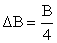and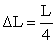for first approximation; thenand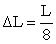for second approximation;andfor third approximation and so on. The ratio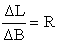is used in graphical presentation and hence it should be kept always the same to make efficient use of graphical representation of FDM expression in computer programming. Some expressions are presented graphically here in Figure 3.Download asVeiw figureFigures index
Figure 2. First approximation nodes of a solid plate
3.3. Solution of FDM Equations by Matrix

Applying the corresponding graphical form of FDM expression at every node will give an individual equation for each node with some unknown deflection components. Therefore, the number of equations will be same as the number of unknown deflection components. A series of equations will be obtained. As number of equations depends on the degree approximation, for better accuracy a large number of equations may be obtained which can be solved easily using matrix method. For this purpose the series of equations are arranged in matrix form. The matrix representation of equations for Figure 2 is shown in Figure 4.

The deflection matrix is obtained by taking inverse of coefficient matrix and multiplying it with load matrix and the constants. This operation can be performed satisfactorily in conjunction with a computer program such as C, C++, FORTAN etc. As deflection matrix is obtained, the plate problem is considered to be solved.

### 4. Analysis of a Sample Plate by FDM

A rectangular steel plate is proposed to analyze by finite difference method. The plate has an internal opening of rectangular shape inside it at its centre. At first the plate without opening is analyzed and then a rectangular opening is considered at its center. Deflections at different points are determined. Moreover, the results obtained by FDM are to be compared with analysis result produced by STAAD.Pro.

4.1. Description of Problem

A rectangular steel plate is subjected to uniformly distributed load by an overlaying concrete slab. The plate is clamped on two opposite edges and free on the other edges. The plate has an internal rectangular opening at its centre. The plate is to be analyzed by finite difference method. Following two cases are to be considered.

a) Considering the plate to be solid.(without opening)

b) Considering the opening.

Following data are given,

1. Length of clamped edge = 8 ft

2. Length of free edge = 12 ft

3. Thickness of plate = 1 inch

4. Thickness of concrete slab = 10 in

5. Opening dimension:

a. Along free edge = 3 ft

b. Along clamped edge = 2ft

6. Unit weight of concretepcf

7. Modulus of elasticity of steel,psi

8. Poisson’s ratio, v = 0.3Download asVeiw figureFigures index
Figure 3. Graphical Representation of FDM expressions (Cont.)Download asVeiw figureFigures index
Figure 4. Matrix form of FDM equations
4.2. Calculation of Parameters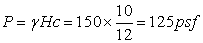Flexural rigidity of slab,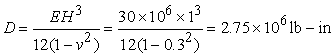Finite difference along fixed edge,The quantity at right hand side of resulting matrix form of FDM equations (Figure 4),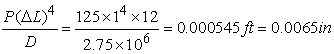4.3. Generation of Nodes

Finite difference nodes are generated by takingand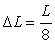as shown in fig. 5

Finite difference along fixed edge,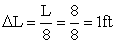The quantity at right hand side of resulting FDM equations.Download asVeiw figureFigures index
Figure 5. Node generation and marking of unknown deflections
4.4. Marking of Unknown Deflection Components

At each node there is one displacement component. There are total 81 nodes on the solid plate. From support consideration all the nodes along the clamped edge have zero deflection. These nodes are not marked as they have zero value. Due to symmetry of loading and structure the nodes at symmetric locations must have equal deflection components and hence they are marked with same deflection notation. Doing this, in total, 20 unknown deflection components are found for the solid plate and 19 unknown deflection components are found for the plate with opening. These are shown in Figure 5.

4.5. Application of FDM Equations at Nodes

The graphical representation of FDM equations are applied at various nodes with unknown deflection components.

For example, at the node with deflection W1 following expression is obtained:

For solid plate, applying Figure 3. (a),(3)

For ease of calculation and convenience of matrix generation these equation can be tabulated as

#### Table 1. Table for equations at point W1

Similar tables are prepared for all the unknown deflection points for both cases, i.e., without and with opening.

4.6. Calculation of Deflection Matrix

The tables prepared in section 4.5 give the equations of deflection in the form,

A11W1+A12W2+A13W3+………+A119W19 =A21W1+A22W2+A23W3+………+A219W19 =A31W1+A32W2+A33W3+………+A319W19 =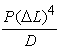… … … … … … … … … … … …

… … … … … … … … … … … …

The matrix representation would take the formTo get the deflection matrix all that we have to do is

I. To inverse the coefficient matrix and

II. Multiplication of the inversed matrix with the right hand side of the above mentioned equation.

### 5. Results from FDM Analysis

After multiplication, the displacement matrix is obtained finally.

For the solid plate,And for the plate with opening,### 6. Comparison of Result with STAAD.Pro Analysis

To verify the feasibility of FDM, the deflection data obtained from the analysis is compared with the deflection data of same plate obtained from a finite element based STAAD.Pro analysis.

Table 2 and Table 3 represents the results obtained from FDM and STAAD.Pro analysis.

#### Table 3. Comparison of node displacements for plate with opening

The comparison tables show that there is a significant variation of deflection result obtained from finite difference analysis and that obtained from STAAD.Pro analysis. When the plate is solid, FDM deflection values are found to be 3.4 % to 28.6% more than corresponding STAAD.Pro deflection values. When the plate is considered to have opening, this variation ranges from 7 % to 27.8 %. It is also found that variation is more at the nodes where deflection is smaller. Minimum variation is found at the node corresponding to maximum deflection. Variation tends to be increased toward the clamped edge.Download asVeiw figureFigures index
Figure 6. Variation of deflection along outer free edgeDownload asVeiw figureFigures index
Figure 7. Variation of deflection along longitudinal middle lineDownload asVeiw figureFigures index
Figure 8. Variation of deflection along transverse middle line

Figure 6, Figure 7 and Figure 8 represent the deflection data comparison graphically. Each of these figures contains four curves; two for plate without opening and two for plate with opening. For each condition node displacement data obtained from FDM analysis and that obtained from STAAD.Pro pakage are plotted against length or width of plate. It is found that the shape of the curves obtained for the FDM data is similar to those obtained for STAAD.Pro results. This indicates validity of FDM for plate analysis. The vertical distance between a FDM curve and its corresponding STAAD.Pro curve indicates the deviation of FDM result from STAAD.Pro solution.

### 7. Conclusion

The finite difference analysis performed here taking 1/8th difference is the second approximation of the exact solution. Therefore, the result obtained is expected to vary with actual result. More accurate result can be obtained by third or fourth approximation taking 1/16th or 1/32th difference. Another way to increase the accuracy of computation is the elimination of error in representation by using Taylor’s expansion. From the result table it is observed that the nodes experiencing maximum and minimum deflection are same for both analyses; they are W4 and W15 respectively. This indicates the accuracy of calculation in FDM analysis. Moreover, deflection pattern is also similar in both cases. Thus, the FDM analysis is found to be satisfactory as compared to STAAD.Pro analysis.

### References

  Cheung M.S., Chan M.Y.T. , Static and dynamic analysis of thin and thick sectorial plates by the finite strip method., Computers and Structures , 14, pp. 79-88, 1981.In article View Article  Fernandes G.R., Venturini W.S. , Stiffened plate bending analysis by the boundary element method., Computational Mechanics, 28, pp. 275-281, 2002.In article View Article  Baltacioglu A.K., Akgoz, B., Civalek, O., Nonlinear static response of laminated composite plates by discrete singular convolution method., Composite Structures, 93, pp. 153-161, 2010.In article View Article  Leissa, A. W. and Niedenfuhr, F. W., Bending of a square plate with two adjacent Edges Free and the others Clamped or simply supported, AIAA Journal, V-1, pp. 116-120, 1962.In article  Aksu G., Ali R., Free vibration analysis of stiffened plates using finite-difference method., Journal of Sound and Vibration, 48, pp. 15-25, 1976.In article View Article  Reddy, J. N., and Gera, R., An Improved Finite-Difference Analysis of Bending of Thin Rectangular Elastic Plates, Comp. Struct., v-10 , pp. 431-438, 1979In article  Vitor M. A. Leitão, A meshless method for Kirchhoff plate bending problems, International Journal for Numerical Methods in Engineering, V-52, I-10, 2001.In article  Yusof Bin Salleh, Mohd., A Finite Difference Simulation of a Flexible Rectangular Plate Structure, thesis submitted to the Faculty of Mechanical Engineering, University Technical Malayasia Malacca., 2007.In article  Ali Ergün and Nahit Kumbasar, A New Approach of Improved Finite Difference Scheme on Plate Bending Analysis, Scientific Research and Essays, Vol. 6(1), pp. 6-17, 4 January, 2011.In article  Szilard, R., Theory and Analysis of Plates, Classical and Numerical Methods, Prentice-Hall, Englewood Cliffs, New Jersey, 1974.In article  Szilard, R., Theories and Applications of Plate Analysis: Classical, Numerical and Engineering Methods, John Wiley & Sons, Inc., United States edition, 2004.In article  Levy, H. and Lessman, F., Finite Difference Equations, Dover Publications, New York., 1992.In article